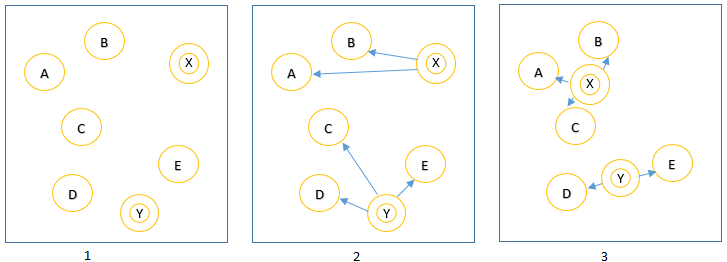# Creating clusters of closely related data, creating k clusters using python

 Category: Machine Learning Tags:K-means clustering code files

## Introduction

K-clustering is a data clustering algorithm which creates k clusters of given data where each cluster has data which is closely related. K is predefined variable which is responsible of how many clusters has to be created.

To create k clusters we will create k centroids and place these at random locations and then we calculate distance of each centroid with each data record. Once we find a record most close to a centroid we associate this data record to centroid once association is done we move this centroid to average position of associated data. We repeat this process until every centroid is placed at its final position as shown in below figure:Fig 1: K-means clustering

Above you can see in figure 1 we placed two centroid X, Y at random locations, figure 2 shows association of X to A, B and Y to C, D, E. And figure 3 shows once X and Y moves to average positions C comes closer to X than Y so we re-associate it with X.

## Implementation

In our previous article we have learned how to create word vectors from given set of text, we took description of companies and identified keywords which can categorize companies. In this article we will use data we produce in last article and saved it in a file called output.json. This JSON file has data formatted as below (This file you can find in attached code):

```{
"Accenture": {
"platform": 0,
"provides": 2,
"government": 0,
"through": 1,
"clients": 0,
"financial": 0,
"services": 2,
"information": 0,
"research": 0,
"health": 0,
"more": 0,
"global": 0,
"solutions": 2,
"technology": 1,
"products": 0,
"analytics": 0,
"software": 0,
"their": 0,
"help": 0,
"from": 0
},...
}```

In this article we are going to create k clusters of above companies, where each cluster has closely related companies. To find distance between data and centroids we will use Pearson correlation score which we have already learned in article here. Just not going back and giving Pearson score function below:

```def pearsonScore(obj1, obj2):
col = {}
for item in obj1:
if item in obj2:
col[item] = 1;
n = len(col)
if(n == 0):
return 0;
#calculating sum
sum1 = sum([obj1[item] for item in col])
sum2 = sum([obj2[item] for item in col])
#calculating sum of squares
sumSq1 = sum([pow(obj1[item], 2) for item in col])
sumSq2 = sum([pow(obj2[item], 2) for item in col])
#calculating sum of products
sumPr = sum([obj1[item] * obj2[item] for item in col])
#calculating pearson score
num = sumPr - (sum1*sum2)/n
den = math.sqrt(abs(sumSq1 - pow(sum1, 2)/n)*abs(sumSq2 - pow(sum1, 2)/n))
if(den == 0):
return 0
return num/den```

Now we have to write method to read JSON file:

```def getCompaniesMatrix():

Now let’s create function kClusterCreate to implement k-means clustering algorithm:

```def kClusterCreate(rows, k, distance_score = pearsonScore):
# picking a company to list all properties
randonCompany = next(iter(rows))
properties = rows[randonCompany]
# getting maximum and minimun values of a keyword
minOfRange = min([row[i] for row in rows.values() for i in properties.keys()])
maxOfRange = max([row[i] for row in rows.values() for i in properties.keys()])
# creating random centroids
centroids = [({item:random.randint(minOfRange, maxOfRange) for item in properties.keys()}) for i in range(k)]
lastMatches = None
for i in range(100):
print("Iteration: ", i)
# array of array to hold cluster
bestMatches = [[] for i in range(k)]
# calculating each centroid distance with a row and assigning a row to centroid which is closer
for key_company, value_company in rows.items():
bestMatch = 0;
for j in range(k):
d = distance_score(value_company, centroids[j])
# centroid and row are more closer if d is higher than last match
if(d > distance_score(value_company, centroids[bestMatch])):
bestMatch = j
# appending company name to centroid which is closest to company
bestMatches[bestMatch].append(key_company)

# if no change in previous calculation and current calculation means we have got our results
if(bestMatches == lastMatches):
break;
lastMatches = bestMatches

# moving centroid to average position
for i_centroid in range(len(centroids)):
rowsOfCentroid = bestMatches[i_centroid]
# creating average data for each centroid
centroids[i_centroid] = {};
for row in rowsOfCentroid:
row_data = rows[row]
for property in properties:
centroids[i_centroid].setdefault(property, 0)
centroids[i_centroid][property] += row_data[property]
# getting average of each property by deviding it by number of rows
for property in properties:
centroids[i_centroid][property] = centroids[i_centroid][property]/len(rowsOfCentroid) if len(rowsOfCentroid) > 0 else 0

return lastMatches```

If we go through line by line with help of comments we can understand above method which has two parts first one creates random k centroids, and the second part has loop up to 100 which again has 3 parts: (1) assigning rows to closest centroid (2) break of main loop if centroids are on their final positions (3) moving centroids to average positions. Now to produce results in JSON file we will write code:

```results = kClusterCreate(getCompaniesMatrix(), 4)
fileName = "company-k-cluster.json"
file = open(fileName, "w")
json.dump(results, file)```

Above code creates file company-k-cluster.json which will have 4 array which is actually clusters of companies, below I have given structure of generated file:

```[
[ "CrowdANALYTIX", "eScholar LLC.", "CB Insights" ...],
[ "Geolytics", "Caspio", "Adobe Digital Government" ...],
[ "Arrive Labs", "BlackRock", "Cambridge Semantics" ...],
[ "Analytica", "Chemical Abstracts Service", "Abt Associates" ...]
]
```

## Conclusion

We can create clusters of data but limitation is we have to provide how many clusters we want to create, this algorithm does not create clusters itself without providing k but this runs better in large datasets.

 Like0 PeopleNikhil Joshi Ceo & Founder at Dotnetlovers Atricles: 133 Questions: 9 Given Best Solutions: 9 *

Login via:x